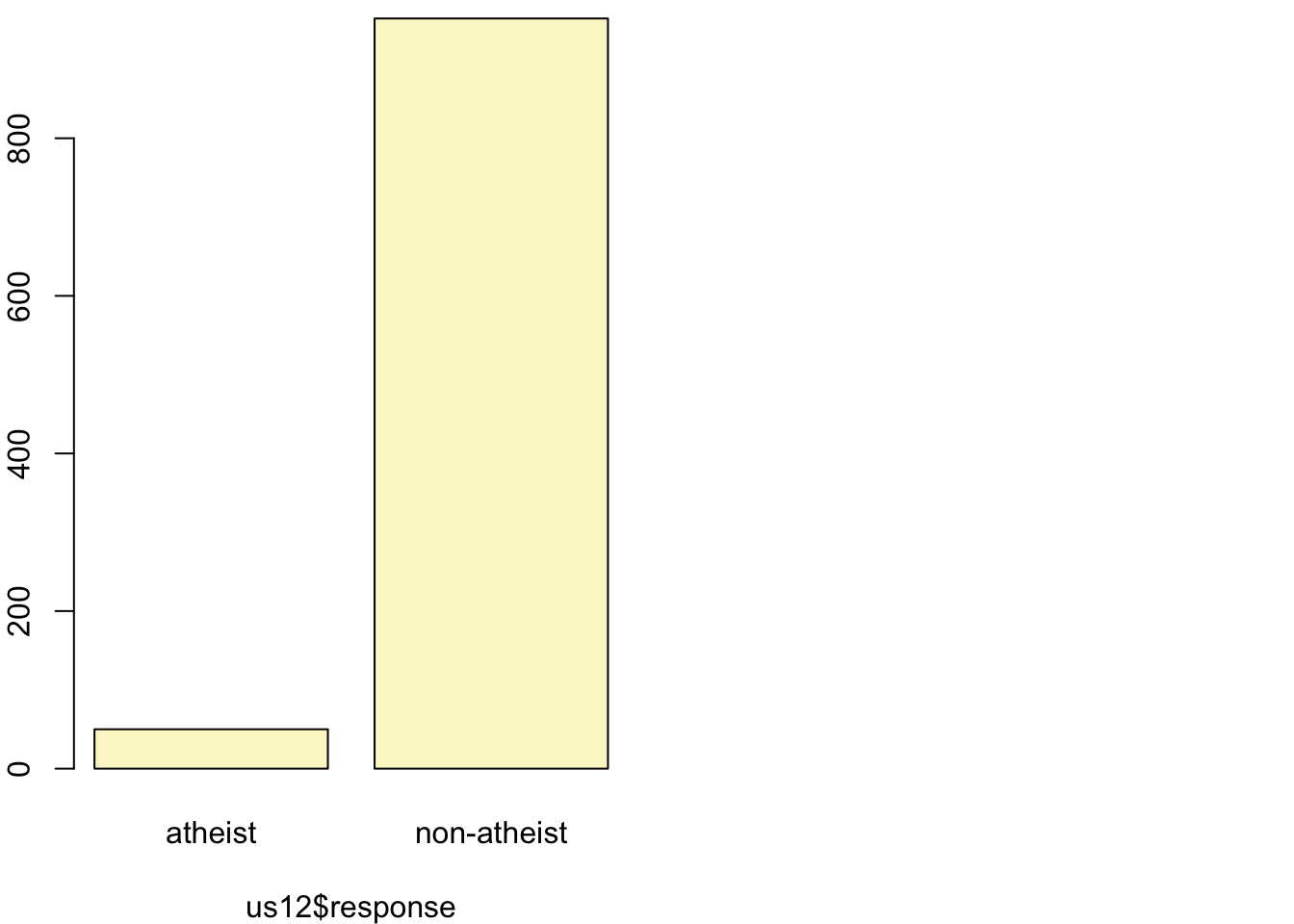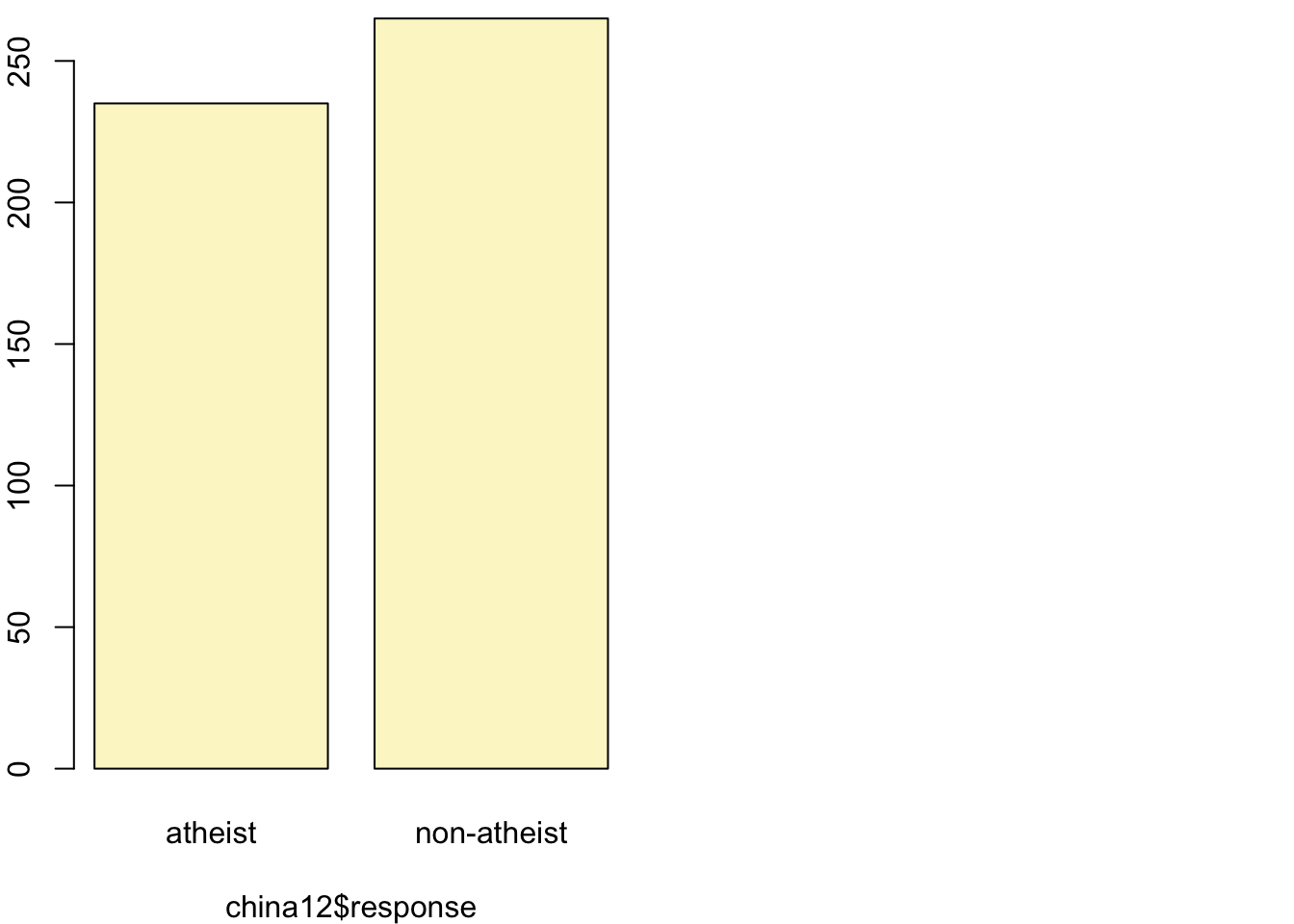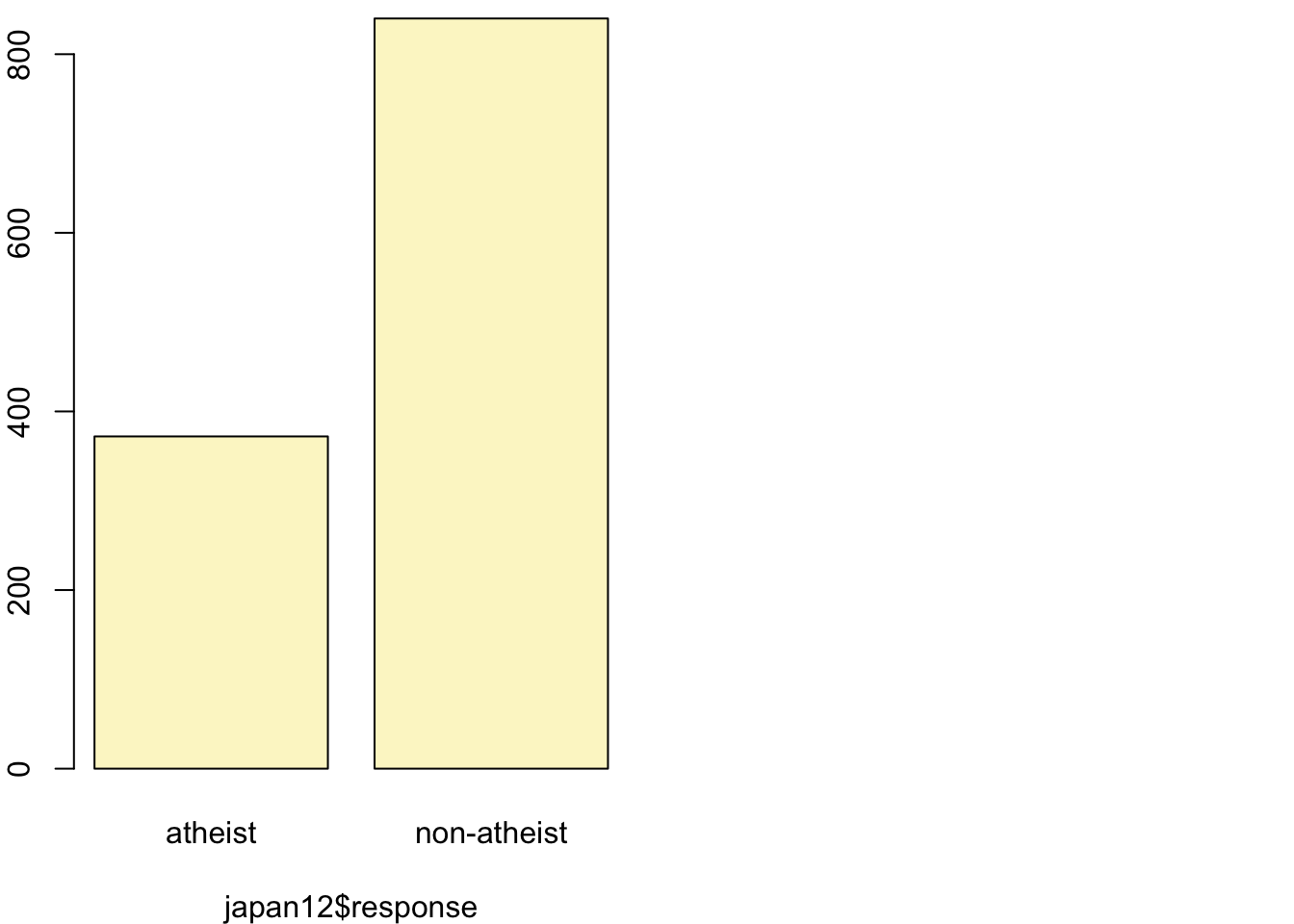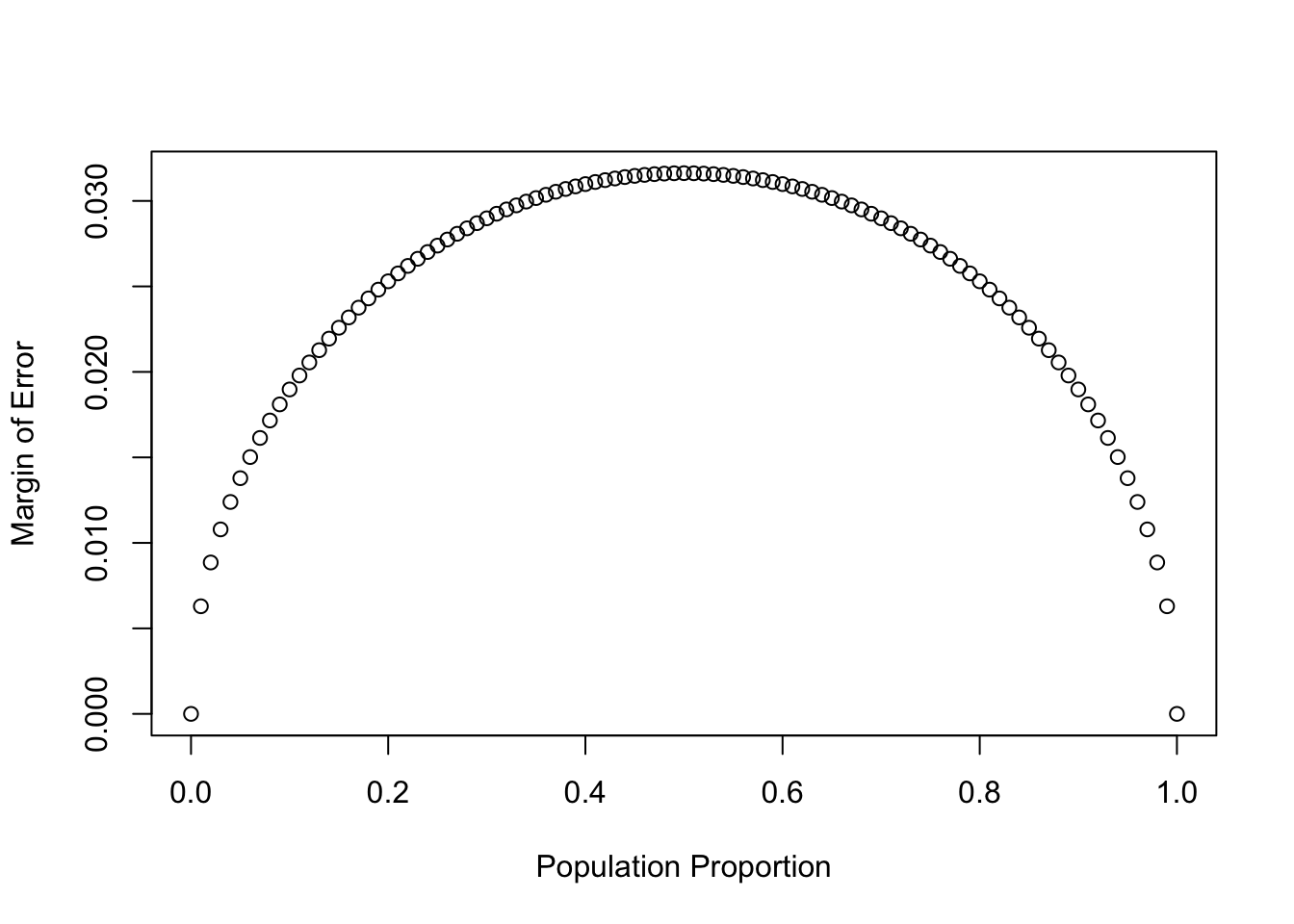In August of 2012, news outlets ranging from the Washington Post to the Huffington Post ran a story about the rise of atheism in America. The source for the story was a poll that asked people, “Irrespective of whether you attend a place of worship or not, would you say you are a religious person, not a religious person or a convinced atheist?” This type of question, which asks people to classify themselves in one way or another, is common in polling and generates categorical data. In this lab we take a look at the atheism survey and explore what’s at play when making inference about population proportions using categorical data.

## The survey

To access the press release for the poll, conducted by WIN-Gallup International, click on the following link:

https://github.com/jbryer/DATA606/blob/master/inst/labs/Lab6/more/Global_INDEX_of_Religiosity_and_Atheism_PR__6.pdf

Take a moment to review the report then address the following questions.

1. In the first paragraph, several key findings are reported. Do these percentages appear to be sample statistics (derived from the data sample) or population parameters?

These percentages are sample statistics, sampled from the global population. Since the entire global population was not surveyed, these are not population parameters.

2. The title of the report is “Global Index of Religiosity and Atheism”. To generalize the report’s findings to the global human population, what must we assume about the sampling method? Does that seem like a reasonable assumption?

We must assume that within groups (countries):
• Each sample was selected randomly (reasonable that each person surveyed was selected randomly)
• Sample subjects are independent (one person’s religion is mostly independent from another person’s religion)
• The sample size is sufficiently large (the survey involves hundreds of people from each country)
• The sample size is no more than 10% of the population (each sample is less than 10% of each country population)

## The data

Turn your attention to Table 6 (pages 15 and 16), which reports the sample size and response percentages for all 57 countries. While this is a useful format to summarize the data, we will base our analysis on the original data set of individual responses to the survey. Load this data set into R with the following command.

load("more/atheism.RData")
1. What does each row of Table 6 correspond to? What does each row of atheism correspond to?

Each row of table 6 corresponds to a country. Each row of “atheism” corresponds to an individual person surveyed.

atheism

To investigate the link between these two ways of organizing this data, take a look at the estimated proportion of atheists in the United States. Towards the bottom of Table 6, we see that this is 5%. We should be able to come to the same number using the atheism data.

1. Using the command below, create a new dataframe called us12 that contains only the rows in atheism associated with respondents to the 2012 survey from the United States. Next, calculate the proportion of atheist responses. Does it agree with the percentage in Table 6? If not, why?

The proportion of of atheist responses in the United States is 0.0499. This agrees with the percentage in table 6.

us12 <- subset(atheism, nationality == "United States" & year == "2012")
nrow(subset(atheism, nationality == "United States" & year == "2012" & response == "atheist")) / nrow(subset(atheism, nationality == "United States" & year == "2012"))
##  0.0499002

## Inference on proportions

As was hinted at in Exercise 1, Table 6 provides statistics, that is, calculations made from the sample of 51,927 people. What we’d like, though, is insight into the population parameters. You answer the question, “What proportion of people in your sample reported being atheists?” with a statistic; while the question “What proportion of people on earth would report being atheists” is answered with an estimate of the parameter.

The inferential tools for estimating population proportion are analogous to those used for means in the last chapter: the confidence interval and the hypothesis test.

1. Write out the conditions for inference to construct a 95% confidence interval for the proportion of atheists in the United States in 2012. Are you confident all conditions are met?

Random: The people surveyed for this dataset are selected randomly.

Sample Size: The sample size for this survey is reasonably large (1002).

Independent: The religion of each person is independent of another person’s religion. Also, the sample size is less than 10% of the population of the United States.

If the conditions for inference are reasonable, we can either calculate the standard error and construct the interval by hand, or allow the inference function to do it for us.

inference(us12$response, est = "proportion", type = "ci", method = "theoretical", success = "atheist") ## ## The downloaded binary packages are in ## /var/folders/7b/rwx99j7116lgz26_t3tx_2hm0000gp/T//RtmpnqWpBl/downloaded_packages ## ## The downloaded binary packages are in ## /var/folders/7b/rwx99j7116lgz26_t3tx_2hm0000gp/T//RtmpnqWpBl/downloaded_packages ## Single proportion -- success: atheist ## Summary statistics:## p_hat = 0.0499 ; n = 1002 ## Check conditions: number of successes = 50 ; number of failures = 952 ## Standard error = 0.0069 ## 95 % Confidence interval = ( 0.0364 , 0.0634 ) Note that since the goal is to construct an interval estimate for a proportion, it’s necessary to specify what constitutes a “success”, which here is a response of "atheist". Although formal confidence intervals and hypothesis tests don’t show up in the report, suggestions of inference appear at the bottom of page 7: “In general, the error margin for surveys of this kind is $$\pm$$ 3-5% at 95% confidence”. 1. Based on the R output, what is the margin of error for the estimate of the proportion of the proportion of atheists in US in 2012? The margin of error is approximately 0.0499 - 0.0364 = 0.0135. 2. Using the inference function, calculate confidence intervals for the proportion of atheists in 2012 in two other countries of your choice, and report the associated margins of error. Be sure to note whether the conditions for inference are met. It may be helpful to create new data sets for each of the two countries first, and then use these data sets in the inference function to construct the confidence intervals. China: Conditions for inference on proportions are met since the samples within China are selected randomly, the sample size for each proportion is large enough (500 total for China, with 235 sucesses and 265 failures and individual people within each country are independent (and the sample sizes are less than 10% of the population of China) Confidence interval for proportion of atheism in China: ( 0.4263 , 0.5137 ) Japan: Conditions for inference on proportions are met since the samples within Japan are selected randomly, the sample size for each proportion is large enough (1212 total for Japan, with 372 sucesses and 840 failures and individual people within each country are independent (and the sample sizes are less than 10% of the population of Japan) Confidence interval for proportion of antheism in Japan: ( 0.281 , 0.3329 ) china12 <- subset(atheism, nationality == "China" & year == "2012") inference(china12$response, est = "proportion", type = "ci", method = "theoretical",
success = "atheist")
## Single proportion -- success: atheist
## Summary statistics:## p_hat = 0.47 ;  n = 500
## Check conditions: number of successes = 235 ; number of failures = 265
## Standard error = 0.0223
## 95 % Confidence interval = ( 0.4263 , 0.5137 )
japan12 <- subset(atheism, nationality == "Japan" & year == "2012")
inference(japan12\$response, est = "proportion", type = "ci", method = "theoretical",
success = "atheist")
## Single proportion -- success: atheist
## Summary statistics:## p_hat = 0.3069 ;  n = 1212
## Check conditions: number of successes = 372 ; number of failures = 840
## Standard error = 0.0132
## 95 % Confidence interval = ( 0.281 , 0.3329 )

## How does the proportion affect the margin of error?

Imagine you’ve set out to survey 1000 people on two questions: are you female? and are you left-handed? Since both of these sample proportions were calculated from the same sample size, they should have the same margin of error, right? Wrong! While the margin of error does change with sample size, it is also affected by the proportion.

Think back to the formula for the standard error: $$SE = \sqrt{p(1-p)/n}$$. This is then used in the formula for the margin of error for a 95% confidence interval: $$ME = 1.96\times SE = 1.96\times\sqrt{p(1-p)/n}$$. Since the population proportion $$p$$ is in this $$ME$$ formula, it should make sense that the margin of error is in some way dependent on the population proportion. We can visualize this relationship by creating a plot of $$ME$$ vs. $$p$$.

The first step is to make a vector p that is a sequence from 0 to 1 with each number separated by 0.01. We can then create a vector of the margin of error (me) associated with each of these values of p using the familiar approximate formula ($$ME = 2 \times SE$$). Lastly, we plot the two vectors against each other to reveal their relationship.

n <- 1000
p <- seq(0, 1, 0.01)
me <- 2 * sqrt(p * (1 - p)/n)
plot(me ~ p, ylab = "Margin of Error", xlab = "Population Proportion")1. Describe the relationship between p and me.

The closer p is to 0.5, the larger the Margin of Error. Population proportion and margin of error have a parabolic relationship.

## Success-failure condition

The textbook emphasizes that you must always check conditions before making inference. For inference on proportions, the sample proportion can be assumed to be nearly normal if it is based upon a random sample of independent observations and if both $$np \geq 10$$ and $$n(1 - p) \geq 10$$. This rule of thumb is easy enough to follow, but it makes one wonder: what’s so special about the number 10?

The short answer is: nothing. You could argue that we would be fine with 9 or that we really should be using 11. What is the “best” value for such a rule of thumb is, at least to some degree, arbitrary. However, when $$np$$ and $$n(1-p)$$ reaches 10 the sampling distribution is sufficiently normal to use confidence intervals and hypothesis tests that are based on that approximation.

We can investigate the interplay between $$n$$ and $$p$$ and the shape of the sampling distribution by using simulations. To start off, we simulate the process of drawing 5000 samples of size 1040 from a population with a true atheist proportion of 0.1. For each of the 5000 samples we compute $$\hat{p}$$ and then plot a histogram to visualize their distribution.

p <- 0.1
n <- 1040
p_hats <- rep(0, 5000)

for(i in 1:5000){
samp <- sample(c("atheist", "non_atheist"), n, replace = TRUE, prob = c(p, 1-p))
p_hats[i] <- sum(samp == "atheist")/n
}

hist(p_hats, main = "p = 0.1, n = 1040", xlim = c(0, 0.18))